# 调试

### 调试预测函数

• 增加训练样本
• 减少样本特征集合
• 增加 feature
• 在已有 feature 基础上，增加多项式作为新的 feature 项(${x}_{1}^{2},{x}_{2}^{2},{x}_{1}{x}_{2},$etc.)
• 尝试减小$\lambda$
• 尝试增大$\lambda$

### 评估预测函数

• 训练集用$\left({x}^{\mathrm{\left(1\right)}},{y}^{\mathrm{\left(1\right)}}\right),\left({x}^{\mathrm{\left(2\right)}},{y}^{\mathrm{\left(2\right)}}\right)...\left({x}^{\mathrm{\left(m\right)}},{y}^{\mathrm{\left(m\right)}}\right)$表示
• 测试集用$\left({x}_{\mathrm{test}}^{\mathrm{\left(1\right)}},{y}_{\mathrm{test}}^{\mathrm{\left(1\right)}}\right),\left({x}_{\mathrm{test}}^{\mathrm{\left(2\right)}},{y}_{\mathrm{test}}^{\mathrm{\left(2\right)}}\right)...\left({x}_{\mathrm{test}}^{\mathrm{\left(mtest\right)}},{y}_{\mathrm{test}}^{\mathrm{\left(mtest\right)}}\right)$表示

1. 使用训练集，求${J}_{train}\left(\Theta \right)$的最小值，得到$\Theta$

2. 使用测试集，计算${J}_{\mathrm{test}}\left(\Theta \right)$

• 对于线性回归函数，计算${J}_{\mathrm{test}}\left(\Theta \right)$的公式为：
${J}_{test}\left(\mathrm{\Theta }\right)=\frac{1}{2{m}_{test}}\sum _{i=1}^{{m}_{test}}\left({h}_{\mathrm{\Theta }}\left({x}_{test}^{\left(i\right)}\right)-{y}_{test}^{\left(i\right)}{\right)}^{2}$
• 对于逻辑回归函数，计算${J}_{\mathrm{test}}\left(\Theta \right)$
${J}_{\mathrm{test}}\left(\Theta \right)=-\frac{1}{{m}_{\mathrm{test}}}\sum _{i=1}^{m}\left[{y}_{\mathrm{test}}^{\mathrm{\left(i\right)}}\mathrm{log}\left({h}_{\theta }\left({x}_{\mathrm{test}}^{\left(i\right)}\right)\right)+\left(1-{y}_{\mathrm{test}}^{\left(i\right)}\right)\mathrm{log}\left(1-{h}_{\theta }\left({x}_{\mathrm{test}}^{\left(i\right)}\right)\right)\right]$
• 对于分类/多重分类问题，先给出错误分类的预测结果为

计算${J}_{\mathrm{test}}\left(\Theta \right)$的公式为：

$\text{Test Error}=\frac{1}{{m}_{test}}\sum _{i=1}^{{m}_{test}}err\left({h}_{\mathrm{\Theta }}\left({x}_{test}^{\left(i\right)}\right),{y}_{test}^{\left(i\right)}\right)$

### 模型选择和训练样本重新划分

1. ${h}_{\mathrm{\left(\theta \right)}}\left(x\right)={\theta }_{0}+{\theta }_{1}x$

（d=1）

2. ${h}_{\mathrm{\left(\theta \right)}}\left(x\right)={\theta }_{0}+{\theta }_{1}x+{\theta }_{2}{x}^{2}$
3. ${h}_{\mathrm{\left(\theta \right)}}\left(x\right)={\theta }_{0}+{\theta }_{1}x+{\theta }_{2}{x}^{2}+{\theta }_{}{x}^{3}$
4. ${h}_{\mathrm{\left(\theta \right)}}\left(x\right)={\theta }_{0}+{\theta }_{1}x+{\theta }_{2}{x}^{2}+{\theta }_{3}{x}^{3}+...+{\theta }_{10}{x}^{10}$

• 训练样本$\left({x}^{\mathrm{\left(1\right)}},{y}^{\mathrm{\left(1\right)}}\right),\left({x}^{\mathrm{\left(2\right)}},{y}^{\mathrm{\left(2\right)}}\right)...\left({x}^{\mathrm{\left(m\right)}},{y}^{\mathrm{\left(m\right)}}\right)$ 占 60%
• 交叉验证样本$\left({x}_{\mathrm{cv}}^{\mathrm{\left(1\right)}},{y}_{\mathrm{cv}}^{\mathrm{\left(1\right)}}\right),\left({x}_{\mathrm{cv}}^{\mathrm{\left(2\right)}},{y}_{\mathrm{cv}}^{\mathrm{\left(2\right)}}\right)...\left({x}_{\mathrm{cv}}^{\mathrm{\left(mvc\right)}},{y}_{\mathrm{cv}}^{\mathrm{\left(mcv\right)}}\right)$占比 20%
• 测试集$\left({x}_{\mathrm{test}}^{\mathrm{\left(1\right)}},{y}_{\mathrm{test}}^{\mathrm{\left(1\right)}}\right),\left({x}_{\mathrm{test}}^{\mathrm{\left(2\right)}},{y}_{\mathrm{test}}^{\mathrm{\left(2\right)}}\right)...\left({x}_{\mathrm{test}}^{\mathrm{\left(mtest\right)}},{y}_{\mathrm{test}}^{\mathrm{\left(mtest\right)}}\right)$占 20%

1. 使用训练集，计算各自的$\Theta$值，用${\Theta }^{\mathrm{\left(d\right)}}$表示
2. 使用验证集，计算各自的${J}_{\mathrm{cv}}\left(\Theta \right)$值，用${J}_{\mathrm{cv}}\left({\Theta }^{\mathrm{\left(d\right)}}\right)$，找到最小值
3. 将上一步得到最小值的 Θ 用测试集验证，得到通用的错误估计值${J}_{\mathrm{test}}\left({\mathrm{\Theta }}^{\left(d\right)}\right)$

### 观察 Bias 和 Variance

• Training error,也就是$J\mathrm{\left(\theta \right)}$,由前面可知，公式为
$J train ( θ ) = 1 2 m ∑ i = 1 m h θ ( x i ) − y i 2$
• Cross Validation error 的公式为
$J cv ( θ ) = 1 2 m cv ∑ i = 1 m cv ( h θ ( x cv ( i ) ) − y cv ( i ) ) 2$

• 对于${J}_{\mathrm{train}}\left(\mathrm{\theta }\right)$（Training Error），当多项式维度 d 增大的时候，错误率逐渐降低
• 对于${J}_{\mathrm{cv}}\left(\theta \right)$（Cross Validation Error），当多项式维度 d 增大到某个值的时候${J}_{\mathrm{cv}}\left(\theta \right)$的值将逐渐偏离并大于${J}_{\mathrm{train}}\left(\mathrm{\theta }\right)$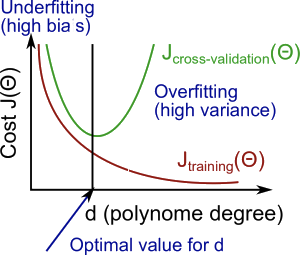• High bias(Underfitting)${J}_{\mathrm{cv}}\left(\theta \right)$的值和${J}_{\mathrm{train}}\left(\mathrm{\theta }\right)$的值都很大，有${J}_{\mathrm{cv}}\left(\mathrm{\theta }\right)\approx {J}_{\mathrm{train}}\left(\mathrm{\theta }\right)$
• High variance(Overfitting)${J}_{\mathrm{cv}}\left(\theta \right)$的值会小于${J}_{\mathrm{train}}\left(\mathrm{\theta }\right)$，在超过某个 d 值后会大于${J}_{\mathrm{train}}\left(\mathrm{\theta }\right)$的值

### Regularization 项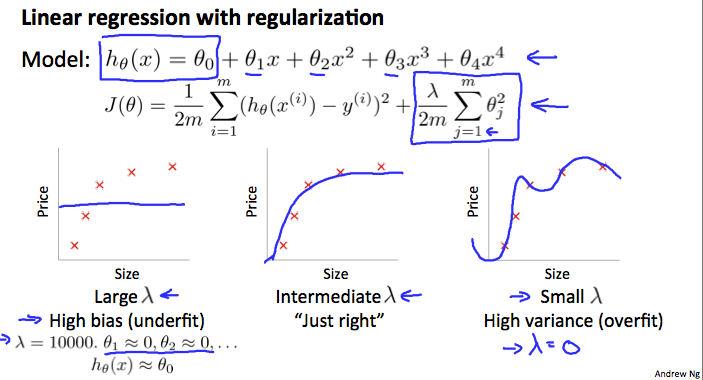1. 先列出一个 λ 数组： (i.e. λ∈{ 0, 0.01, 0.02, 0.04, 0.08, 0.16, 0.32, 0.64, 1.28, 2.56, 5.12, 10.24 })
2. 创建一系列不同 degree 的预测函数
3. 遍历 λ 数组，对每一个 λ 带入所有 model，求出$\Theta$
4. 将上一步得到的$\Theta$，使用交叉验正数据集计算${J}_{\mathrm{cv}}\left(\Theta \right)$，注意${J}_{\mathrm{cv}}\left(\Theta \right)$是不带 regularization 项的，或者 λ=0
5. 选出上面步骤中使${J}_{\mathrm{cv}}\left(\Theta \right)$得到最小值的$\Theta$和 λ
6. 将上一步选出的$\Theta$和 λ 使用 test 数据集进行验证，看预测结果是否符合预期

### 绘制学习曲线

• High bias - 样本数少：${J}_{\mathrm{train}}\left(\Theta \right)$ 值很低，${J}_{\mathrm{cv}}\left(\Theta \right)$值很高 - 样本数大：${J}_{\mathrm{train}}\left(\Theta \right)$${J}_{\mathrm{cv}}\left(\Theta \right)$都很高，当样本数超过某个值时，有${J}_{\mathrm{train}}\left(\Theta \right)$${J}_{\mathrm{cv}}\left(\Theta \right)$ - 如果某个学习算法有较高的 bias，仅通过增加训练样本是没用的 - 学习曲线图如下所示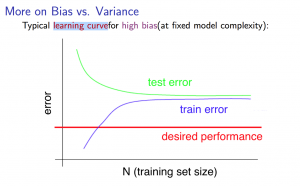• high variance - 样本数少：${J}_{\mathrm{train}}\left(\Theta \right)$ 值很低，${J}_{\mathrm{cv}}\left(\Theta \right)$值很高 - 样本数大：${J}_{\mathrm{train}}\left(\Theta \right)$ 升高，但还是保持在一个较低的水平。 ${J}_{\mathrm{cv}}\left(\Theta \right)$则持续降低，但还是保持在一个较高的水平，因此有${J}_{\mathrm{train}}\left(\Theta \right)$ < ${J}_{\mathrm{cv}}\left(\Theta \right)$。两者的差值很大 - 如果某个学习算法有较高的 variance，通过增加训练样本有可能有帮助 - 学习曲线图如下所示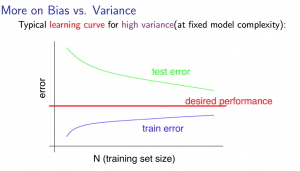### 调试预测函数(Revisit)

• 增加训练样本数量 - 解决 high variance 问题
• 减少样本特征数量 - 解决 high variance 问题
• 增加样本特征 - 解决 high bias 问题
• 增加多项式维度 - 解决 high bias 问题
• 减小 λ 值 - 解决 high bias 问题
• 增大 λ 值 - 解决 high variance 问题

#### 调试神经网络

• 一个层数少，每层 unit 个数少的神经网络，容易 underfitting，计算成本很低
• 一个层数多，每层 unit 个数也很多的神经网络，容易 overfitting，计算成本也很高，这种情况可加入 regularization 项（增大 λ）来尝试解决

## Machine Learning System Design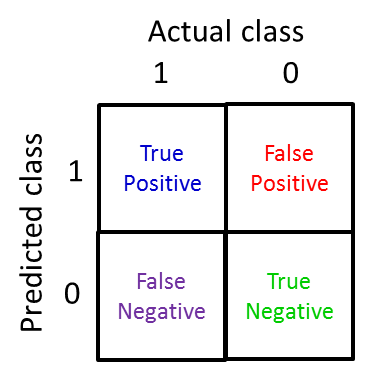• 如何收集足够多的高质量训练数据，广告邮件，垃圾邮件等，这些训练数据的来源该去哪里获得
• 选取哪些作为关键的特征，比如邮件中出现”discount”,”deal”等，但是这些可能会误杀一些邮件，出了这些是否还是其它的信息可作为 feature
• 如何设计出全面严谨的模型，比如有些垃圾邮件会故意把某些单词拼错(e.g. m0rtage, med1cine, w4tches 等)来绕过反垃圾检查，训练模型要能识别出这些错误的拼写

1. 先从一个简单的算法开始，快速实现，在验证样本集中进行验证
2. 绘制学习曲线，观察是否需要增加训练样本或者增加特征
3. 进行误差分析，例如，在验证集中找出那些分类不准的 email，查看出错原因，观察能否找到一些共性或者系统性的错误

• Error Metrics for Skewed CLasses

• 准确率(Precision)：precision = #(true positives)/#(predicted positives)
• 召回率(Recall): recall = #(true positives)/#(actual positives)

$hθ(x) >= threshold$

$F1 Score : 2 PR P+R$

F 分数越大说明 threshold 计算出的值越合理，通常我们使用交叉验证样本集算出一个较好的 threshold 再使用测试集验证结果